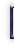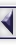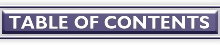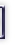# Numerical Workbenches, part III

#### By Christoph Spiel

Parts I and II of this little series on numerical math workbenches on GNU/Linux have covered rather dry subjects like matrix manipulations and for-loops. This part brings more color to the screens as it turns to the graphical capabilities of GNU/Octave, Scilab, and Tela. It will deviate from the previous way of presenting the three applications, because the differences of the graphical backends are far too big to allow for a uniform treatment with only a few explanations on the differences.

The article starts with an introduction applicable to all programs. To give the reader maximum benefit, all applications have to solve the same three real life problems. This simplifies the comparison despite the differences of the implementations. In the last part, Octave, Scilab, and Tela have to tackle the given tasks.

## Introduction

Discrete Data Only
As all three applications are designed to work with vectors and matrices, their graphics backends only understand this kind of data. Doh! For the user this means that the graph of a mathematical function like
```                { sin(x)/x  for x <> 0
y := f(x) = {
{    1      for x == 0
```

cannot be drawn from the above expression, but the function has to be transformed into discrete pairs (x(i)y(i)). This transformation is called sampling. To sample f(x), we pick values x where we are interested in f(x) and compute f for the given x. Any reader who has followed the earlier parts, will immediately recognize that this involves a vector operation.

```    ### GNU/Octave code
```
```    function y = f(x)
if x == 0.0
y = 1.0;
else
y = sin(x) ./ x;
endif
endfunction
```
```    x = linspace(0.0, 4*pi, 50);
y = f(x);
```

linspace(start, end, n) returns a vector from start to end, whose n elements are evenly spaced. The vectors x and y can be passed to an appropriate plotting function.

If data already are in vectorized form, they can be displayed immediately.

Complexity at Large
How do we actually display a graph? This question leads us to the fundamental problem all applications run into when it comes to graphical output of data: either complicated or many simple function calls! Let us first consider the complexity for the user of a call to solve a system of linear equations
```    x = a \ b             # Octave, Scilab
```

or

```    x = linsolve(a, b)    // Tela
```

Even if we try hard to artificially complicate the calls (yet leaving them useful), we hit the end of the road at

```    x = linsolve(a, b, "CompletePivoting", "DoIterativeRefinement")
```

This is, with the two additional parameters -- pivoting strategy and iterative refinement of the solution -- the user completely controls linsolve(). All other ``decisions'' can reasonably be taken by the workbench itself, for example, what algorithm to use if matrix a has a special form.

Contrast this with the 2d-graph of

```    x = [2.25,  2.27,  2.42,  ...]
y = [0.363, 0.360, 0.337, ...]
```

What options can we think of?

• Draw isolated data points or connect data points with straight lines.
• Which symbol to draw at each data point? A dot, star, circle, or asterisk? What color should the data points be?
• Which kind of line to draw between the data points? Continuous, dashed, dotted, or dash-dotted? What line thickness and what color is the line?
• Scale the plot to fit within the plotting window?
• Draw coordinate axes? Linear or logarithmic x or y axis? Both axes logarithmic?
• Graduate or annotate the axes?
• ...

Many more reasonable options are conceivable. The point is: we cannot expect plotting to be as simple to use as is for example solving a system of linear equations -- not because the applications are badly written, but because the topic is inherently more complicated on the user's side. Hence, nobody should be surprised if she ever needs 20 or more lines to define a publication quality plot in Octave, Scilab, or Tela.

## Problems

To make the competition interesting, I put up problems, which are not too far away from the Real World(tm). Each of the three applications will have to cope with three different types of plots:

1. 2D Plot of Discrete Data

Display three sets of data (l1.ascii, l2.ascii, l3.ascii) on a single page. The sets have a different number of data points.

The data are stored as two columns, column 1 holds the x-value, and column 2 the y-value:

```    0.2808  3.419E-07
0.3711  3.459E-07
0.4882  3.488E-07
...
```

The graph must show title, axes captions, and legend. The x and y ranges are supplied by the user.

2. 3D Plot of a Function
Plot Rosenbrock's function (in two dimensions)
```    f(u, v) = 100*(v - u^2)^2 + (1 - u)^2
```

as a 3d-surface in the parameter ranges -3 <=  u <= 3 and -2 <=  v <= 4.

Annotate the plot with title and axes captions.

3. Contour Plot of a Function
Plot function
```    g(u, v) = exp(u) * (4*u^2 + 2*v^2 + 4*u*v + 2*v + 1)
```

with contours, this is, f(u, v) = z for given z in the parameter ranges -4.5 <= u <= -0.5 and -1 <= v <= 3.

The iso-lines are defined by a user-specified ``weight'' function.

```                          (  z - z_0  ) 3
z_iso = (z_1 - z_0) * ( --------- )   + z_0
( z_1 - z_0 )
```

where z_0 and z_1 are the minimum and maximum values of g(u, v) in the plot's range.

Again, annotate the plot with title and axes captions.

All graphics must be rendered for two devices,

• X11 terminal and
• Postscript printer.

## Octave

You know Gnuplot? Then learning to use GNU/Octave's plot functions will be a piece of cake. All you need to do is precede the Gnuplot commands with a ```g`'' to reach at the Octave equivalent. -- Oh, you haven't tried Gnuplot yet? Then I will guide you through the examples. However, having a Gnuplot manual (online or dead-tree edition) ready may be helpful.

If you do not want to download Gnuplot's manual, you can use one of the publicly accessible versions.

### Octave: 2D Discrete Data Plot

For an easier discussion, I have split the code into blocks. Each block gathers commands that belong together. The blocks are labeled with numbers in square brackets, to allow for referencing them in the text.

```    ###  Read data set_i into N_i-times-2 matrices
```
```    ###  Reset Gnuplot
graw("reset;");
clearplot;
```
```    ###  Define decorations and plot area
gset title "Comparison of sets L1, L2, and L3";
gset xlabel "Temperature / K";
gset ylabel "Voltage / V";
gset key top left;
gset xrange [0 : 100];
gset yrange [8e-8 : 2e-6];
```
```    ###  Plot data
hold on;
gplot set1 title "Set L1" with points;
gplot set2 title "Set L2" with points;
gplot set3 title "Set L3" with points;
hold off;
```
```    ###  Switch to PostScript output and plot into file
gset terminal push;
gset terminal postscript;
gset output "oct1.eps";
replot;
gset terminal pop;
gset output;
```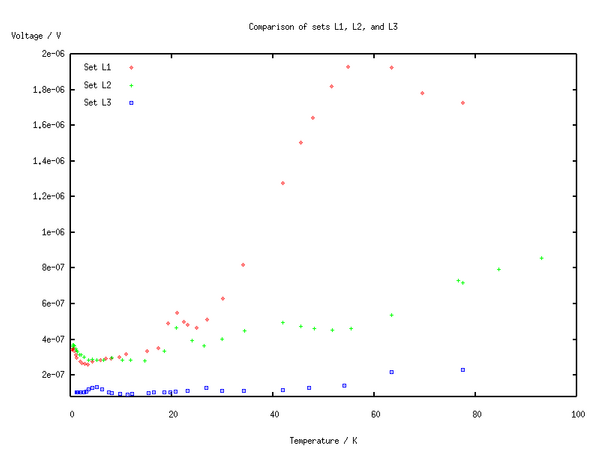The output to the Postscript terminal, block , produced a printable version (eps) of the graphics.

Block  should be clear from the previous articles. The first interaction with Gnuplot happens in , where Gnuplot is reset to a known state and the X11-plot window gets cleared. A known state is helpful when still experimenting with Gnuplot's options. In our case reseting is unnecessary, but it does not hurt.

The block of `gset` commands,  is self explaining except

```    gset key top left
```

which means: ``put the legend (or key) inside the plotting area at the north-west corner''. `xrange` and `yrange` with their special interval syntax set the width and height of the plot range.

Now, the actual data can be rendered into the plot window . Because the data sets have different sizes, they cannot be gathered in a single matrix. Hence, each data set must be plotted by itself, and Gnuplot must be told -- with `hold on` -- that it should collect all ``` gplot``` commands until the last plot of the set is completed and `hold off` is called. The calls to gplot essentially contain the data matrix

```    gplot set1
```

The title, which shows up in the plot's legend, is assigned in the call to `gplot`, too.

Block  is specific to the way Gnuplot works. For each plot it uses a terminal and an output file (remember that in UN*X everything is a file, as for example is the console). When Gnuplot is running under X, the terminal type defaults to X11 and output file defaults to Gnuplot's X11-graphic window. Both, terminal and output file can be changed independently with `gset` commands. Thus, to get the same graphics in a different format, we switch from the current terminal to Postscript, and put an ordinary file's name at the output. Function `replot` replays all plot commands for us, we do not have to write them again. After that the terminal and output settings are undone, which is optional, but helpful if the user wants to twiddle some more `gset`'s and repeatedly check their influence on the graph's appearance.

### Octave: 3D Function Plot

```    ###  Define function
function z = f(u, v)
## truncated Rosenbrock function
z = 100.0*(v - u.^2).^2 + (1.0 - u).^2;
zv = z(:);
zv(find(zv > 100.0)) = 100.0;
z = reshape(zv, size(z));
endfunction
```
```    ###  Sample function f()
x = linspace(-3, 3, 40);
y = linspace(-2, 4, 40);
[xx, yy] = meshgrid(x, y);
z_splot = splice_mat(xx, yy, f(xx, yy));
```
```    ###  Reset Gnuplot
graw("reset;");
clearplot;
```
```    ###  Define decorations and viewing direction
gset data style line;
gset title "Rosenbrock Function";
gset xlabel "u";
gset ylabel "v";
gset view 30, 160;
gset hidden;
gset nokey
gset parametric;
```
```    ###  Plot
gsplot z_splot;
```
```    ###  Switch to PostScript output and plot into file
gset terminal push;
gset terminal postscript;
gset output "oct2.eps";
replot;
gset terminal pop;
gset output;
gset noparametric;
```
```    system("gzip --best --force oct2.eps");
```A printer-ready version (eps.gz) is available, too.

Again the first block, , should be easy to understand for anyone who has read Part 1 and 2 of this series for it only uses known functions. In contrast,  introduces two new functions. Out friend linspace() quickly generates two vectors which will define where f(u, v) will be evaluated: from vectors x and y, a grid is constructed, this is, two matrices xx and yy, where each pair of matrix elements (xx(i, j), yy(i, j)), 1 <= ij <= 40, define a (grid-) point at which function z = f(u, v) will be evaluated. The matrix of all z-values at the grid points then simply is ```zz = f(xx, yy)```. However, we are not done at this point, because Octave requires to pass a specially formatted matrix to the 3d-plot function gsplot.

The user-defined function splice_mat() does exactly the needed operation: collect the grid data xx and yy along with the z-values in matrix  z_plot. Matrix z_plot can be handed over to gsplot without further ado (given Gnuplot is in parametric mode. (we will be in parametric mode and for the given kind of problem parametric mode is the only way to go (and I don't want to introduce another level of parenthesis (really!))))

Block , a collection of `gset`s, looks almost familiar. Some new settings are

• gset data style line
Join adjacent mesh-points with (by default: red) lines.
• gset view x_angle, z_angle
Rotate the view point around the x-axis by x_angle degrees, then rotate around the new z-axis by z_angle degrees.
• gset hidden
Suppress invisible mesh lines. The back side of the mesh is drawn differently (green in the X11-window and with dashed black lines in the Postscript terminal).
• gset nokey
Suppress any legend.
• gset parametric
See GNU/Octave's documentation. (`help -i gsplot` from Octave, or `info octave --node='Three-Dimensional Plotting'` from the shell.)

After so much preparation, the actual plot command, ```gsplot z_splot``` in  looks trivial.

The Postscript block  is similar to  in Section ``Octave: 2d discrete data plot''. The only additional job  does is gzipping the eps-file. In general system(``shell-commands'') executes shell-commands in a sub-shell. Obviously, this is extremely useful when interacting with an external application, like gzip(1).

### Octave: Contour Function Plot

```    ###  Define function
function z = g(u, v)
z = exp(u) .* (4.0*u.^2 + 2.0*v.^2 + 4.0*u.*v + 2.0*v + 1.0);
endfunction
```
```    ###  Define weight function for iso-line distances
function y = pow_weight(x, n)
## Map interval X onto itself, weight with N-th power.
d = max(x) - min(x);
y = d*((x - min(x))/d).^n + min(x);
endfunction
```
```    ###  Sample function g()
x = linspace(-4.5, -0.5, 40);
y = linspace(-1.0, 3.0, 40);
[xx, yy] = meshgrid(x, y);
zz = g(xx, yy);
z_splot = splice_mat(xx, yy, zz);
```
```    ###  Compute iso-line distances
iso_levels = pow_weight(linspace(min(min(zz))*1.01, ...
max(max(zz))*0.99, 12), 3.0);
il_str = sprintf("%f,", iso_levels);
il_str = il_str(1 : length(il_str)-1);    # remove last ","
```
```    ###  Reset Gnuplot
graw("reset;");
clearplot;
```
```    ###  Define decorations and viewing direction
gset data style line;
gset title "Contour Plot of g(u, v)";
gset xlabel "u";
gset ylabel "v";
gset contour base;
gset nosurface;
gset view 0, 0;
eval(sprintf("gset cntrparam levels discrete %s", il_str));
gset parametric;
```
```    ###  Plot
gsplot z_splot;
```
```    ###  Switch to PostScript output and plot into file
gset terminal push;
gset terminal postscript;
gset output "oct3.eps";
replot;
gset terminal pop;
gset output;
gset noparametric;
```A printable version (eps) is available, too.

After working through the 2d discrete and the 3d function plot examples, the top three blocks [1-3] of the contour function script should not raise many questions. In block  however, I have to prepare a trick, which I must play at the end of block . The job is to plot contour lines with a user-defined function. The values of this function and therefore the positions of the iso-lines are not known in advance.

Gnuplot offers several ways to define iso-lines, for example, automatic computation of a given number of (linearly spaced) contours or defining a minimum and a maximum contour value with a fixed increment between two adjacent contours. Our problem requires a more general solution because the iso-lines are not equally spaced along the z-axis. For completely arbitrary iso-line values, as the third way if specifying contour lines, Gnuplot has the following `gset` command

```    gset cntrparam discrete z1, z2, ..., zN
```

where z1, z2, ..., zN are the z-values of the iso-lines given as floating point literals. Thus,

```    gset cntrparam discrete 0.2, 0.4, 0.8
```

is a perfect call, whereas

```    z1 = 0.2
z2 = 0.4
z3 = 0.8
gset cntrparam discrete z1, z2, z3
```

is nothing but a syntax error as would be

```    gset cntrparam discrete iso_levels
```

Remember, `gset` needs floating point literals!

We are stuck unless we apply a bit of magic. If we present a complete `gset` line to Octave, one where the values of vector iso_levels are ``interpolated'' into (Perl programmers do this all day long), the race is won. Here is how the trick is played:

```    # Convert iso_levels to comma-separated string.  Octave reuses
# the format specifier list if there are more items to print than
# there are format specifiers.  Don't try this with C's printf :-)
il_string = sprintf("%f,", iso_levels)
```
```    # Remove comma past last contour value in the string.
il_string = il_string(1 : length(il_string)-1)
```
```    # Play interpolation trick a second time
gset_string = sprintf("gset cntrparam levels discrete %s", il_string);
```
```    # Execute the command which is stored in the variable gset_string
eval(gset_string);
```

For readers who do not like abstract descriptions, this is a transcript of a session (where long lines have been edited to fit):

```    octave:10> il_string = sprintf("%f,", iso_levels)
il_string = 0.583444,0.592029,0.652120,0.815224,1.132847,1.656497, \
2.437679,3.527900,4.978667,6.841486,9.167864,12.009307,
octave:11> il_string = il_string(1 : length(il_string)-1)
il_string = 0.583444,0.592029,0.652120,0.815224,1.132847,1.656497, \
2.437679,3.527900,4.978667,6.841486,9.167864,12.009307
octave:12> gset_string = sprintf("gset cntrparam levels discrete %s", \
il_string)
gset_string = gset cntrparam levels discrete 0.583444,0.592029, \
0.652120,0.815224,1.132847,1.656497,2.437679,3.527900, \
4.978667,6.841486,9.167864,12.009307
```

In the script, no temporary variable gset_string has been introduced, but sprintf() sends its output directly to eval().

Block : Gnuplot does not excel in plotting contours. In fact the GNuplot user-manual suggests not to use it directly. Anyhow, we proceed, because the out approach is easier to understand. The following three ``` gset```s switch Gnuplot into contour-mode:

```    gset contour base    # draw contours in the xy-plane
gset nosurface       # do not draw the surface's mesh
gset view 0, 0       # view the xy-plane from above
```

Blocks  and  closely resemble those we have already seen in Section ``Octave: 2D Discrete Data Plot'' and ``Octave: 3D Function Plot''.

More demo plots of Gnuplot can be found at http://www.gnuplot.org/gnuplot/gpdocs/all2.htm

## Scilab

And now for a rather different approach ...

Scilab goes a different way to cope with the complexity, which we have discussed in Section Complexity at Large. In contrary to Gnuplot, Scilab does not strictly separate plotting and setting, but offers a plethora (buckle up, then try: ```apropos plot```) of different plot commands to produce different kinds of plots. Furthermore, the plot functions themselves take many arguments that change the plots' appearances. Some of the arguments are so cryptic that you almost certainly will want to have the appropriate man-page within sight.

For the reader who wants to look at the on-line help, but does not have Scilab installed, the Scilab manual is available online.

### Scilab: 2D Discrete Data Plot

```    //  Read data set_i into N_i-times-2 matrices
```
```    //  Clear plot window's contents
xbasc();
```
```    //  Plot data; 1st plot command defines plot area
plot2d(set1(:, 1), set1(:, 2), -1, "011", ..
rect = [0, 8e-8, 100, 2e-6]);
plot2d(set2(:, 1), set2(:, 2), -2, "000");
plot2d(set3(:, 1), set3(:, 2), -3, "000");
```
```    //  Define decorations
xtitle(["Comparison of sets", "L1, L2, and L3"], ..
"Temperature / K", "Voltage / V");
legends(["Set L1   ", "Set L2   ", "Set L3   "], [-1, -2, -3], 2);
```
```    //  Save plot window's contents to file; convert file to PostScript
xbasimp(0, "sci1.xps");
unix("scilab -save_p sci1.xps.0 Postscript");
```The Encapsulated Postscript output is available, too.

Block  reads the data from disk-files into matrices.

Block  clears the graphics window (if it exists). Even more important, xbasc() deletes all graphics commands that have been recorded for the window. The mnemonic for xbasc() is x-bas-c for x11-function, basic level, clear.

Tip: When playing with Scilab's plot functions, preceding the plot function call with a clear command often saves keystrokes

```    xbasc(); plot2d(...);
```

in one line can be recalled for editing with a single C-p and replayed with a single hit of the return-key.

In Block  the enigma raises its head! What in the world does

```   plot2d(set1(:, 1),                      // vector of x-values
set1(:, 2),                      // vector of y-values
-1,                              // style index
"011",                           // decoration control
rect = [0, 8e-8, 100, 2e-6]);    // plot dimensions
```

do? I have added a comment for each argument, yet, to what does a ``style index'' of -1 refer to? A badly dressed hacker? And what does the string in ``decoration control'' mean?

Style index
controls the kind of marker to draw for each data point or the color of the line that joins the data points. A positive value i means: draw a line with color i, a negative value means: use marker type abs(i). Note that plot2d() draws either markers or lines, never both. If markers and lines are required for the same data set, two plots with different style indices are necessary.
Decoration control
always is a string of length 3. Each character of the string controls a certain aspect of the plot's decorations.
Character 1
Toggle display of the plot caption. '`0`' is no caption, '`1`' tells Scilab to display a caption.
Character 2
Control how the size of the plot is computed. Valid characters range from '`0`' to '`8`'. Please see plot2d() documentation for details.

Here, we use '`1`' for the first plot and '`0`' for all others. '`1`' lets the user explicitely specify the plot range in the `rect` argument to plot2d(). If plot2d() is called with '`0`', the size of a previous plot is used and no size computation is done.

Character 3
Set the type of axes drawn around the plot. The user can choose from six different values. We choose '`1`' for the first plot, which gives axes on the bottom and the left hand side. The following plots use '`0`', which means nothing is drawn around the plot.

Gee, that is quite a mouthful! Block  will give us some rest.

```    xtitle([title_line1; title_line2; ...], x_label, y_label)
```

Puts a possibly multi-line title into an existing plot and optionally decorates the x and y-axis with labels.

```    legends([legend_1, legend_2, ...], [style_1, style_2, ...], position)
```

Places a legend in a plot where the position is given by position. position = 1 refers to the north east corner. The remaining corners are enumerated counter-clockwise, this is, position = 2 will put the legend in the north west corner. The style_i parameters refer to the same numbers that we have used as style indices in the plot2d() calls. They determine which kind of marker or line is drawn right next to the legend's text. The text is given by legend_i.

Finally, Block  converts the data in the graphics window to a Postscript file.

```    xbasimp(0, "sci1.xps")
```

Replays all graphics commands for window 0 (our only graphics window) into sci1.xps. The function's name has nothing to do with a X11-daemon named ``bas'', but has its stem from x11 and basic level as xbasc(). The ``imp'' comes from the French word ``imprimer'', which means ``to print''. From which language does ``fsck'' come from? N vcls, I guess.

File sci1.xps almost contains valid Postscript, but not quite. The contents must be processed by Scilab

```    scilab -save_p sci1.xps.0 Postscript
```

adding the correct Postscript header and repositioning the graphics on the page. The external Scilab is called by the unix(``shell-commands'') function.

### Scilab: 3D Function Plot

```    //  Define function
function z = f(u, v)
// truncated Rosenbrock function
z = 100.0*(v - u.^2).^2 + (1.0 - u).^2
z(find(z > 100)) = 100;
endfunction
```
```    //  Define sampling grid for f()
x = linspace(-3, 3, 40);
y = linspace(-2, 4, 40);
```
```    //  Clear plot window's contents
xbasc();
```
```    //  Plot
fplot3d(x, y, f, 65, 1.5);
```
```    //  Define decoration
xtitle("Rosenbrock Function", "u", "v");
```
```    //  Save plot window's contents to file; convert file to PostScript
xbasimp(0, "sci2.xps");
unix("scilab -save_p sci2.xps.0 Postscript; " ..
+ "gzip  --best --force sci2.eps");
```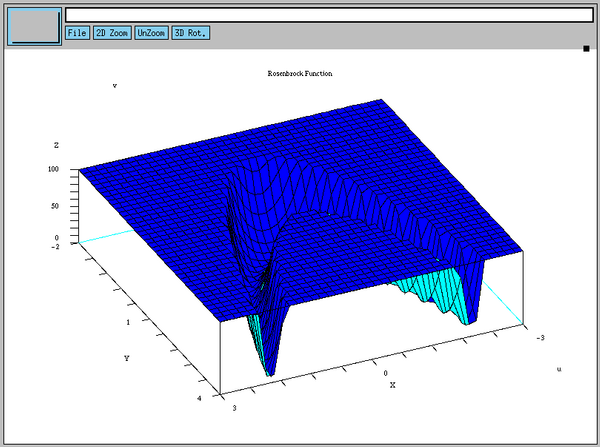Here is the gzipped Encapsulated Postscript version (eps.gz) of the graphics.

After so much new stuff in Section Scilab: 2D Discrete Data Plot the only unknown here hides in Block :

```    fplot3d(x_vector, y_vector, function_of_x_and_y, alpha, theta)
```

The vectors x_vector and y_vector define the grid on which function_of_x_and_y is evaluated. Comparing fplot3d() with Octave's gsplot we notice, that Scilab generates the grid for us. Function fplot3d() is a convenience function, built on top of

```    plot3d(x_vector, y_vector, z_matrix, alpha, theta)
```

which resembles gsplot (and Tela's mesh()).

The parameters theta and alpha, define the elevation of the view point and the rotation around the z-axis respectively.

### Scilab: Contour Function Plot

```    //  Define function
function z = g(u, v)
z = exp(u) .* (4.0*u.^2 + 2.0*v.^2 + 4.0*u.*v + 2.0*v + 1.0)
endfunction
```
```    //  Define weight function for iso-line distances
function y = pow_weight(x, n)
// Map interval X onto itself, weight with N-th power.
d = max(x) - min(x)
y = d*((x - min(x))/d).^n + min(x)
endfunction
```
```    //  Define sampling grid for g()
x = linspace(-4.5, -0.5, 40);
y = linspace(-1.0, 3.0, 40);
```
```    //  Evaluate g() at points defined by X and Y
z = eval3d(g, x, y);
```
```    //  Compute iso-line distances
iso_levels = pow_weight(linspace(min(z)*1.01, max(z)*0.99, 12), 3.0);
```
```    //  Clear plot window's contents
xbasc();
```
```    //  Set format of iso-line annotation and plot
xset("fpf", "%.2f");
contour2d(x, y, z, iso_levels);
```
```    //  Define decoration
xtitle("Contour Plot of g(u, v)", "u", "v");
```
```    //  Save plot window's contents to file; convert file to PostScript
xbasimp(0, "sci3.xps");
unix("scilab -save_p sci3.xps.0 Postscript");
```The output is available as printer-ready version (eps), too.

Remember the difficulties we had with the user-defined iso-lines in Octave/Gnuplot? The problem can be solved in Scilab without any tricks, magic, or bribery. As with plot3d(), Scilab defines a convenience function

```    fcontour2d(x_vector, y_vector, function_of_x_and_y, levels)
```

as a wrapper around

```    contour2d(x_vector, y_vector, z_matrix, levels)
```

However, as we must know the maximum and minimum value of g(u, v) on the grid, fcontour2d() would not save us anything. Thus, Block  computes g(u, v) on the grid defined by x and y:

```    z = eval3d(g, x, y)
```

In Block , we use z and iso_levels from Block . The xset() call sets the floating point format (``fpf'') to ```%.2f`'', which is a C-printf format specifier that forces all contour line numbers to have two digits after the decimal point.

All other blocks only use functions already discussed in the other plots.

More demo plots of Scilab are found at INRIA's Scilab site.

Scilab has much more to offer than the functions we have seen right above. For example, it supplies

• Low-level functions to draw points, lines, polygons, etc.
• Surface facets which are the building blocks for 3d-environments. (See for example Enrico Segre's amazing Scigallery)

• High-level GUI-interface for rapid development of tailor-made Scilab applications.
• Feedback functions that allow passing back information from any graphics window to Scilab.

## Tela

In the sections on Octave and Scilab, we have seen that both applications save auxiliary ``state'' of the plot window. Gnuplot does so to the max: `gplot` or ``` gsplot``` have almost no parameters. The state is controlled by ``` gset``` commands. Scilab stores some state of the plot window as, for example, the legend and title.

Tela uses the external application PlotMTV to display graphs. With PlotMTV, Tela is located on the other side of the spectrum: its plots have no state, and all information about the plot has to go into the call to the actual plotting function. The advantage of Tela's approach is that multiple plots do not interfere (unless we force them to do so by calling `hold(on)` or `hold(off)` as we will see soon). The downside is that the calls to plotting functions can get rather long.

As has been in the discussions of the other plotting applications, it is helpful to have a PlotMTV manual within reach.

### Tela: 2D Discrete Data Plot

```    //  Read data set_i into N_i-times-2 matrices
set1 = import1("l1.ascii");
set2 = import1("l2.ascii");
set3 = import1("l3.ascii");
```
```    //  Define plotting function
function do_plot(d1, d2, d3)
{
hold(on);    // postpone actual plotting until hold(off)
```
```         // render set 1
plot(d1[:, 1], d1[:, 2],
"linestyle",  0,
"markertype", 2,
"linelabel",  "Set L1",
// Define decorations
"toplabel",   "Comparison of sets",
"subtitle",   "L1, L2, and L3",
"xlabel",     "Temperature / K",
"ylabel",     "Voltage / V",
// Define plot area
"xmin",       0,
"xmax",       100,
"ymin",       8e-8,
"ymax",       2e-6);
// render set 2
plot(d2[:, 1], d2[:, 2],
"linestyle",  0,
"markertype", 3,
"linelabel",  "Set L2");
// render set 3
plot(d3[:, 1], d3[:, 2],
"linestyle",  0,
"markertype", 4,
"linelabel",  "Set L3");
```
```         hold(off);    // plot!
};
```
```    //  Plot to X11 window
do_plot(set1, set2, set3);
```
```    //  Plot into a postscript file
plotopt("-o tela1.eps -printcmd 'cat' -noxplot -print");
do_plot(set1, set2, set3);
```

Hmm, this script looks differently! It does -- because I have to play another trick that has to do with the statelessness plot functions. As Tela ``forgets'' the parameters after displaying graphics in an X11-window, I would have to retype everything with output redirected to a Postscript file. Well, code duplication is known to be the root of many (not all) evil. Thus, the Tela plots are wrapped into a function. The further strategy is displaying the plot in an X11 window, changing the output file and type, which is somewhat analogous to Gnuplot, and then plotting again into an eps-file:

```    do_plot(...);    // display plot in X11 window
plotopt("-o foo.eps -printcmd 'cat' -noxplot -print");  // redirect output
do_plot(...);    // render plot in Encapsulated Postscript file foo.eps
```Encapsulated Postscript version of the graphics.

Function do_plot in Block  takes care of all output by using function plot(). The general structure of Tela's plot(), in fact all Tela plot functions, is first passing the mandatory data arguments as vectors or matrices followed by optional key-value strings.

```    plot(x_vector, y_vector                 // data
"option_key1", "option_value1",    // option 1
"option_key2", "option_value2",    // option 2
...
"option_keyN", "option_valueN");   // option N
```

Note that putting one key-value pair per line enhances the readability of the whole call.

The key-value strings themselves are self-explaining. Non-obvious associations like linestyle => 0 (= invisible line) or markertype => 2 (= plus sign) must be looked up in the manual or in a reference plot which shows all available line styles.

### Tela: 3D Function Plot

```    function v = linspace(a, b; n)
{
if (isdefined(n)) nn = n else nn = 100;
v = a + (0 : nn - 1) * (b - a) / (nn - 1)
};
```
```    //  Define function
function z = f(u, v)
{
// truncated Rosenbrock function
z = 100.0*(v - u^2)^2 + (1.0 - u)^2;
z[find(z > 100.0)] = 100.0;
};
```
```    //  Sample function f()
x = linspace(-3.0, 3.0, 40);
y = linspace(-2.0, 4.0, 40);
[xx, yy] = grid(x, y);
zz = f(xx, yy);
```
```    //  Define plot function
function do_plot(x, y, zz)
{
mesh(zz,
"xgrid",       x,
"ygrid",       y,
"toplabel",    "Rosenbrock Function",
"xlabel",      "u",
"ylabel",      "v",
"hiddenline",  "true",
"eyepos.z",    2.0)
};
```
```    //  Render plot into X11 window
do_plot(x, y, zz);
```
```    //  Plot into a postscript file
plotopt("-o tela2.eps -printcmd 'cat' -noxplot -print");
do_plot(x, y, zz);
system("gzip --best --force tela2.eps");
```An gzipped Encapsulated Postscript version (eps.gz) of the plot is available, too.

Tela has no built in linspace() function, so I quickly define one.

Block  resembles Block  in Section ``Octave: 3D Function Plot'', where Octave's meshgrid() has been replaced by Tela's grid().

The 3d-mesh plotting function mesh() takes a matrix of z-values as its first argument; the grid-specification is given in the options:

```        mesh(z_matrix,
"xgrid", x_vector,
"ygrid", y_vector);
```

Of course, the sizes of z_matrix, x_vector, and y_vector must be compatible.

### Tela: Contour Function Plot

```    function v = linspace(a, b; n)
{
if (isdefined(n)) nn = n else nn = 100;
v = a + (0 : nn - 1) * (b - a) / (nn - 1)
};
```
```    //  Define function
function z = g(u, v)
{
z = exp(u) * (4.0*u^2 + 2.0*v^2 + 4.0*u*v + 2.0*v + 1.0)
};
```
```    //  Define weight function for iso-line distances
function y = pow_weight(x, n)
{
// Map interval X onto itself, weight with N-th power.
d = max(x) - min(x);
y = d*((x - min(x))/d)^n + min(x)
};
```
```    //  Sample function f()
x = linspace(-4.5, -0.5, 40);
y = linspace(-1.0, 3.0, 40);
[xx, yy] = grid(x, y);
zz = g(xx, yy);
```
```    //  Compute iso-line distances
iso_levels = pow_weight(linspace(min(zz)*1.01, max(zz)*0.99, 12), 3.0);
il_str = sformat("``", iso_levels);
il_str = il_str[2 : length(il_str)];
```
```    //  Define plot function
function do_plot(x, y, zz, iso_levels_str)
{
contour(zz,
"xgrid",    x,
"ygrid",    y,
"toplabel", "Contour Plot of g(u, v)",
"xlabel",   "u",
"ylabel",   "v",
"contours", iso_levels_str)
};
```
```    //  Render plot into X11 window
do_plot(x, y, zz, il_str);
```
```    //  Plot into a postscript file
plotopt("-o tela3.eps -printcmd 'cat' -noxplot -print");
do_plot(x, y, zz, il_str);
```Also available is an Encapsulated Postscript printer version.

Tela requires us to play a similar trick on PlotMTV as we had to when convincing Octave to pass the contours' values to Gnuplot. However, the trick in Block  is not as involved. Again, the first step is to compute the vector iso_levels. Function sformat() works analogously to Octave's sprintf(), though the vectors are differently formatted as the transcript shows (il_str has been edited to fit the line).

```    >il_str = sformat("``", iso_levels);
>il_str
"#(0.583444, 0.592029, 0.65212, 0.815224, 1.13285, 1.6565, \
2.43768, 3.5279, 4.97867, 6.84149, 9.16786, 12.0093)"
```

PlotMTV does understand a vector that is enclosed in parenthesis, but the leading sharp-sign must go

```    il_str = il_str[2 : length(il_str)];
```

In this form, il_string can be passed as value to the option key `contours` in function contour().

More demo plots of PlotMTV can be found at ORNL and at HSC.

By the way, PlotMTV suits perfectly as graphical backend for home grown scripts and programs. The PlotMTV manual consistently helps the reader to develop programmatic interfaces for her own applications.

####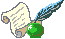Christoph Spiel